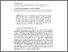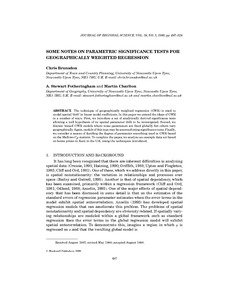Some notes on parametric significance tests for geographically weighted regression

Brunsdon, Chris and Fotheringham, Stewart and Charlton, Martin (1999) Some notes on parametric significance tests for geographically weighted regression. Journal of Regional Science, 39 (3). pp. 497-524. ISSN 0022-4146Previewmore...Add this article to your Mendeley library

Abstract

The technique of geographically weighted regression (GWR) is used to model spatial ‘drift’ in linear model coefficients. In this paper we extend the ideas of GWR in a number of ways. First, we introduce a set of analytically derived significance tests allowing a null hypothesis of no spatial parameter drift to be investigated. Second, we discuss ‘mixed’ GWR models where some parameters are fixed globally but others vary geographically. Again,models of this type may be assessed using significance tests.Finally, we consider a means of deciding the degree of parameter smoothing used in GWR based on the Mallows C p statistic. To complete the paper, we analyze an example data set based on house prices in Kent in the U.K. using the techniques introduced.

Item Type: Article parametric significance tests; geographically weighted regression; Faculty of Science and Engineering > Research Institutes > National Centre for Geocomputation, NCG 5990 Martin Charlton 26 Mar 2015 10:46 Journal of Regional Science Wiley-Blackwell YesItem control page